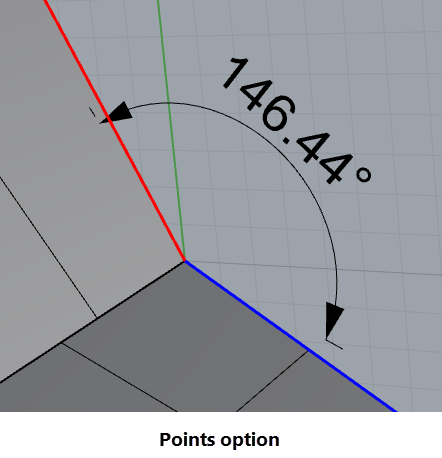# Cannot get a correct measure for angle

Hello everyone,

I’m trying to measure and annotate (dimangle) the angle between two faces of one side of my object. And repeat this operation for the 4 other sides.
I only tried with the first side, but I cannot seem to get a coherent value. The command will write down wierd values (190°, 33°,…).

Could you please have a look?
You can refer to attached images and file.

thanks!

Lampe 03 02.3dm (6.5 MB)

Hi @cgepner, use `_DimCreaseAngle`.

also, in general, Rhino dimensions work in the active CPlane of the current viewport. To measure the angles of the surface edges, you’ll need to set the CPlane properly before creating the dimension.
_
c.

Try DimCreaseAngle?

thanks @clement
could you please explain what cplane i should set in my specific case?
I tried setting one of the two surfaces (that i’m trying to get an angle out of) but it didn’t seem to work
thanks

Hi @cgepner,

for `_DimCreaseAngle`, you do not have to set a CPlane and usually for measurements you could do this without changing CPlane. To measure the angle of the border edges (of the 2 touching planar surfaces), you might just extract the edge lines using `_DupEdge`, make sure they are linear (your’s where not, i used `_SimplifyCrv` on them) and while keeping both lines selected, run `_Angle` and choose the `_TwoObjects` command line option to print the angle.

For drawing angle dimensions, CPlane should be set. In your case you might use `_CPlane _3Point` and click the origin where the edges meet, then `X` and `Y` somewhere on the edge as shown below. Then you can make eg. an arc which you can pick with the `_DimAngle` command.

To set back the CPlane, use `_CPlane _Undo`.,.
_
c.

1 Like

To measure the angle between two planar surfaces without creating a dimension use Agnle with the TwoObjects option.
Angle then the TwoObjects option
Select two planar surfaces.

@davidcockey, if i try this with the model posted and pick the surfaces, i get:

`Angle = 33.564`

obviously, the reflex angle.

@pascal, i think this thread is a wonderful example to show where the angle measurement and angle dimension commands could be improved. It is not possible to measure properly by picking sub-objects and using them with the `TwoObjects` option (prints nothing when edges are picked) nor does `_DimAngle` work using the `_Points` option, it always constrains to World.Top.
_
c.

2 Likes

Angle with TwoObjects provides the smaller angle (0 to 90 degrees) between two planes independent of the normals of the planes.

A geometry fundamental. The angle between the edges of two surfaces will not equal the angle between the surfaces unless the edges are normal to the intersection line of the two surfaces (extended if needed to intersect).

Yeah, I see that…

so is the main thing to look at the Angle> TwoObjects command? Edges (Sub-objects) may return different numbers than curves duped from the same edges…

-Pascal

1 Like

Hi @Pascal, yes for the `_Angle` command using `_TwoObjects`option i would have expected that with a post-selection of the two linear edges it should print an angle.

For the `_DimAngle` command, i would have expected to have the angular dimension drawn in the plane defined by the 3 points picked with the `_Points` option instead of the world xy plane.

_
c.

1 Like

I don’t know… I get what you’re after here and it makes sense in a way, but in general Rhino dims are only CPlane based. (I do not see that it is using World, here, but current CPlane…?)

-Pascal

As far as I can see this one works (V6&7…)

-Pascal

Hi @Pascal, if you set the CPlane as shown in my image above, then use `_DimAngle` and pick the the first line (polysurface edge), do you see that nothing happens and it keeps asking for first line ?

_
c.

1 Like

Hi Clement - OK, I see `DimAngle` not working there - yes…but Points works… are we on the same page?
https://mcneel.myjetbrains.com/youtrack/issue/RH-56812

-Pascal

Hi Pascal, i see `_Angle _TwoObjects` and `_DimAngle _Points` both fail in V6. Using `_Angle` command, there is no angle printed when picking the edges as sub-objects after choosing the `_TwoObjects` option:

Using the `_DimAngle` and `_Points` option, the CPlane it draws the angular dimension in is parallel to world xy with the origin picked at the first point:

I can only draw the angular dimension properly if i set my CPlane as shown in above image. Imho, 3 Points define a plane, so why not use them or add a new option to the command to draw in the point’s plane ?

_
c.

weird - I get different results in both cases

an angle from Angle > TwoObects…

Command: Angle
TwoObjects
Angle = 33.564

(Edges Ctrl-Shift selected…)

and-Pascal

Even when i set my CPlane as yours it does not print an angle here…tried to pick curves and polysurface edge…

_
c.

Hi Clement - have you tried in V7? My 6 is certainly newer than yours - not that I am aware of any changes that would affect this - but those changes would be in V7, presumably.

@clement - Here’s my test file…
DimAngle_SubObjects.3dm (2.2 MB)

-Pascal

Hi Pascal,

even with our file i get no angle printend on `_Angle _TwoObjects` after picking the red and blue curve. If i use `_SimplifyCrv` on them, it works. (6.22.20028.13281, 28.01.2020) on Win7

`_Dim_Angle` creates a dimension using the `_Points` option.
_
c.